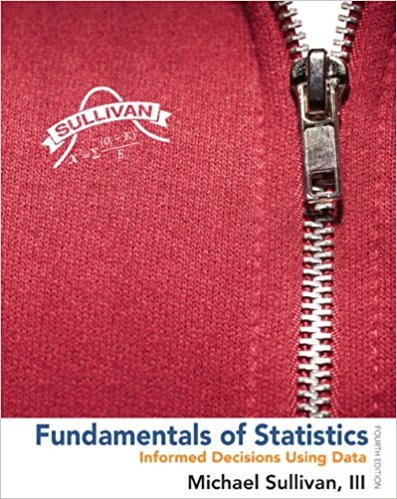×
×

# Determine whether the normal probability plot indicatesISBN: 9780321838704 30

## Solution for problem 3AYU Chapter Chapter 7.3

Fundamentals of Statistics | 4th Edition

• Textbook Solutions
• 2901 Step-by-step solutions solved by professors and subject experts
• Get 24/7 help from StudySoup virtual teaching assistantsFundamentals of Statistics | 4th Edition

4 5 1 308 Reviews
21
2
Problem 3AYU

Determine whether the normal probability plot indicates that the sample data could have come from a population that is normally distributed.Step-by-Step Solution:
Step 1 of 3Step...

Step 2 of 3

Step 3 of 3

##### ISBN: 9780321838704

This textbook survival guide was created for the textbook: Fundamentals of Statistics, edition: 4. This full solution covers the following key subjects: ayu, come, could, data, determine. This expansive textbook survival guide covers 51 chapters, and 2146 solutions. Fundamentals of Statistics was written by and is associated to the ISBN: 9780321838704. Since the solution to 3AYU from Chapter 7.3 chapter was answered, more than 233 students have viewed the full step-by-step answer. The answer to “Determine whether the normal probability plot indicates that the sample data could have come from a population that is normally distributed.” is broken down into a number of easy to follow steps, and 21 words. The full step-by-step solution to problem: 3AYU from chapter: Chapter 7.3 was answered by , our top Statistics solution expert on 04/03/17, 08:02AM.

Unlock Textbook Solution